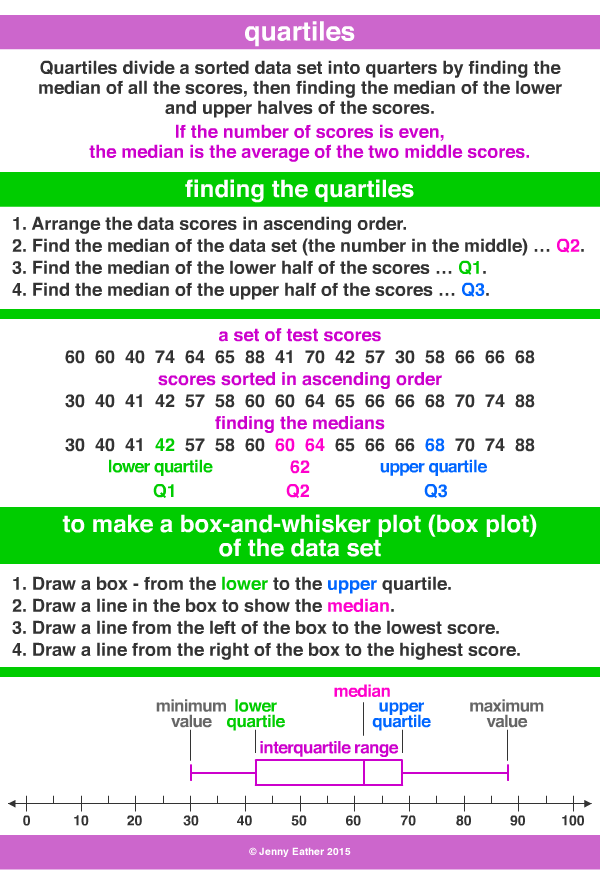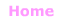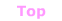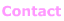Aa Bb Cc Dd Ee Ff Gg Hh Ii Jj Kk Ll Mm Nn Oo Pp Qq Rr Ss Tt Uu Vv Ww Xx Yy Zz

Qq

quartiles

• quartiles are found using medians to
divide a data set into quarters.
• The median is middle value.
• Quartile 1 (Q1) is the median of the lower half of the values.
• Quartile 3 (Q3) is the median of the upper half of the values.
• a box-and-whisker plot or box plot using a number line
is often used to display the minimum value, lower quartile,
median, upper quartile and maximum value.

EXAMPLES:© Jenny Eather 2014. All rights reserved.